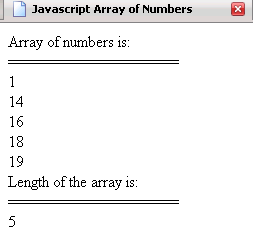Tutorials

# JavaScript Array of number

In this Tutorial we want to describe you a code that help you in understanding Java Script array number of elements.

In this Tutorial we want to describe you a code that help you in understanding Java Script array number of elements.

# JavaScript Array of number

In this Tutorial we want to describe you a code that help you in understanding Java Script array number of elements. We are using JavaScript as script language. We declare an array variable that is used to store array object. An array component  is accessed by an array expression that consists of an expression whose value is an array reference indicated by subscripts indexing expression enclosed by []. The document. write print the Array of numbers followed by line splitter. The for loop run the script till the variable i is less than the array length. The document. write print the list of array element that array object hold in it and also print the length of an array.

javascriptarraynumberofelements.html

 ```      Javascript Array of Numbers            var array=new Array();   array=1;   array=14;   array=16;   array=18;   array=19;   document.write("Array of numbers is: "+"
");   document.write("==================="+"
");   for(var i=0;i");   }   document.write("Length of the array is: "+"
");   document.write("==================="+"
");     document.write(array.length+"
");       ```

Output of the program